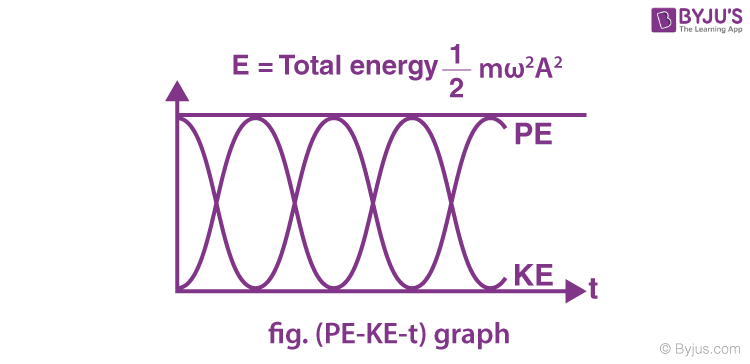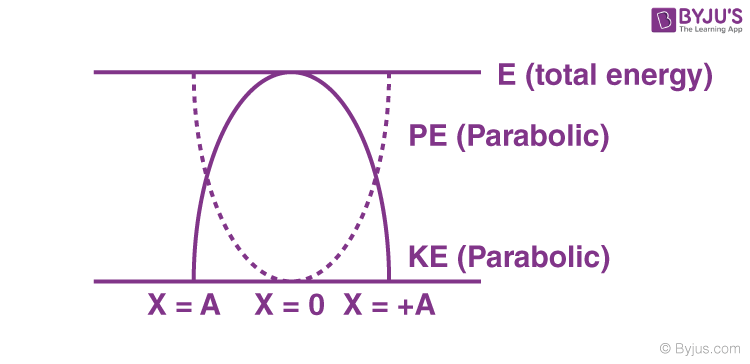# Energy In Simple Harmonic Motion

Energy is a quantitative property of the matter, which is the key factor for performing the work. Energy is defined as the ability to do work. Energy is the fundamental unit for many types of motions. Energy, depending on the intensity, can lead to deformations too. According to the laws of conservation of energy, it is stated that “energy cannot be created or destroyed, although it can be changed from one form to another”. The international system of units of measurement of energy is Joule.

There are many types of energy. Many objects store energy in order to perform work. Batteries store chemical and potential energy. Biomass and petroleum store chemical energy. Mechanical energy is stored in the stretched rubber bands. Solar energy is an example of radiant energy. Rock on the top of a hill stores gravitational potential energy. Lightning stores electrical energy.

Examples: Gasoline undergoes combustion reaction inside the car’s engine in order to operate the car. Here chemical energy is converted into mechanical energy. Solar cells convert light energy into electrical energy.

We can observe in the above examples that one form of energy is converted into another form and is not wasted. Energy is stored for a purpose in various bodies, objects and devices.

Through the following ways, energy can be transferred:

• By Radiation – By Light waves or Sound waves
• Mechanically – By the action of force
• Electrically – Electrically
• By Heating – By conduction, convection, or radiation

## Oscillations and Simple Harmonic Motion

Oscillations are repetitive variations that are periodic. Oscillations are measured about a point of equilibrium, also known as central value or between two or more different states. Repeating variations of any quantity or measure about its equilibrium value in time is defined as oscillation. Oscillations also occur in dynamic systems or, more accurately, in every field of science.

Example: The movement of a simple pendulum in a clock.

Simple Harmonic Motion or SHM is defined as a motion in which the restoring force is directly proportional to the displacement of the body from its mean position. SHM is a special case of oscillation in which motion takes place along a straight line between the two extreme points. A restoring force is seen directed towards the mean position or towards the equilibrium position in SHM. The mean position in simple harmonic motion is a stable equilibrium.

Note: All the SHM are oscillatory and periodic.

Every oscillatory motion is not a simple harmonic motion.

Let us know the energy in simple harmonic motion.

## Energy In Simple Harmonic Motion

We can note there involves a continuous interchange of potential and kinetic energy in a simple harmonic motion. The system that performs simple harmonic motion is called the harmonic oscillator.

Case 1: When the potential energy is zero, and the kinetic energy is a maximum at the equilibrium point where maximum displacement takes place.

Case 2: When the potential energy is maximum, and the kinetic energy is zero, at a maximum displacement point from the equilibrium point.

Case 3: The motion of the oscillating body has different values of potential and kinetic energy at other points.

Consider a particle of mass m, executing linear simple harmonic motion of <a href=”https://byjus.com/physics/period-angular-frequency/”>angular frequency</a> (ω) and amplitude (A) the displacement

$$\begin{array}{l}\left( \overrightarrow{x} \right),\end{array}$$
velocity
$$\begin{array}{l}\left( \overrightarrow{v} \right)\end{array}$$
and acceleration
$$\begin{array}{l}\left( \overrightarrow{a} \right)\end{array}$$
at any time t are given by

x = A sin (ωt + Φ)

v =

$$\begin{array}{l}A\omega \cos \left( \omega t+\phi \right)=\omega \sqrt{{{A}^{2}}-{{x}^{2}}}\end{array}$$

a =

$$\begin{array}{l}-{{\omega }^{2}}A\sin \left( \omega t+\phi \right)=-{{\omega }^{2}}x\end{array}$$

The restoring force

$$\begin{array}{l}\left( \overrightarrow{F} \right)\end{array}$$
acting on the particle is given by

F = -kx

Where k = mω<sup>2</sup>.

### Kinetic Energy of a Particle in Simple Harmonic Motion

Kinetic Energy =

$$\begin{array}{l}\frac{1}{2}m{{v}^{2}}\end{array}$$
$$\begin{array}{l}\left[ Since, \;{{v}^{2}}={{A}^{2}}{{\omega }^{2}}{{\cos }^{2}}\left( \omega t+\phi \right) \right]\end{array}$$

=

$$\begin{array}{l}\frac{1}{2}m{{\omega }^{2}}{{A}^{2}}{{\cos }^{2}}\left( \omega t+\phi \right)\end{array}$$

=

$$\begin{array}{l}\frac{1}{2}m{{\omega }^{2}}\left( {{A}^{2}}-{{x}^{2}} \right)\end{array}$$

Therefore, the Kinetic Energy =

$$\begin{array}{l}\frac{1}{2}m{{\omega }^{2}}{{A}^{2}}{{\cos }^{2}}\left( \omega t+\phi \right)=\frac{1}{2}m{{\omega }^{2}}\left( {{A}^{2}}-{{x}^{2}} \right)\end{array}$$

### Potential Energy of Simple Harmonic Motion

Work done by the restoring force while displacing the particle from the mean position (x = 0) to x = x:

The work done by restoring force when the particle has been displaced from the position x to x + dx is given by

dw = F dx = -kx dx

w =

$$\begin{array}{l}\int{dw}=\int\limits_{0}^{x}{-kxdx=\frac{-k{{x}^{2}}}{2}}\end{array}$$

=

$$\begin{array}{l}-\frac{m{{\omega }^{2}}{{x}^{2}}}{2}\end{array}$$
$$\begin{array}{l}\left[ \,k=m{{\omega }^{2}} \right]\end{array}$$

=

$$\begin{array}{l}-\frac{m{{\omega }^{2}}}{2}{{A}^{2}}{{\sin }^{2}}\left( \omega t+\phi \right)\end{array}$$

Potential Energy = -(work done by restoring force)

Potential Energy =

$$\begin{array}{l}\frac{m{{\omega }^{2}}{{x}^{2}}}{2}=\frac{m{{\omega }^{2}}{{A}^{2}}}{2}{{\sin }^{2}}\left( \omega t+\phi \right)\end{array}$$

### Total Mechanical Energy of the Particle Executing Simple Harmonic Motion

The total energy of the system of a block and a spring is equal to the sum of the potential energy stored in the spring plus the kinetic energy of the block and is proportional to the square of the amplitude.

$$\begin{array}{l}\frac{1}{2}m{{\omega}^{2}}\left({{A}^{2}}-{{x}^{2}}\right)+\frac{1}{2}m{{\omega}^{2}}{{x}^{2}}\end{array}$$

E =

$$\begin{array}{l}\frac{1}{2}m{{\omega }^{2}}{{A}^{2}}\end{array}$$

Hence, the total energy of the particle in SHM is constant and it is independent of the instantaneous displacement. Relationship between potential energy, kinetic energy, and time in Simple Harmonic Motion at t = 0, when x = ±A.Variation of kinetic energy and potential energy in SHM with displacement:Stay tuned with BYJU’S for more such interesting articles on physics, chemistry and maths in an engaging way with video explanations.

## Frequently Asked Questions on Simple Harmonic Motion

Circular motion.

### 2. Give an example of periodic motion.

Motion of a pendulum.

Periodic motion.

### 4. State laws of conservation of energy.

It states that “energy cannot be created or destroyed, although it can be changed from one form to another”.

### 5. Give an example for simple harmonic motion.

Oscillation of Simple Pendulum.